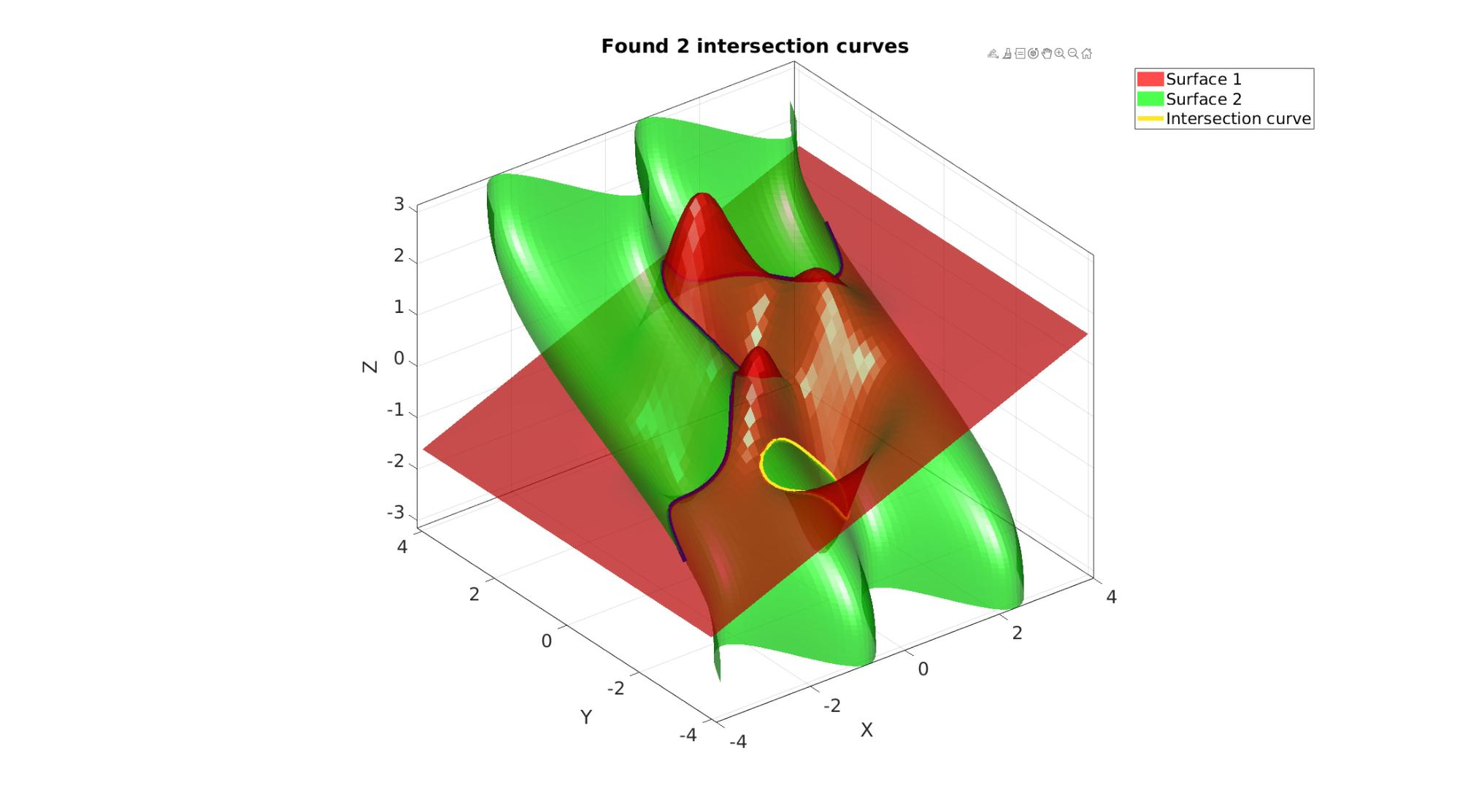# surfacePairIntersect

Below is a demonstration of the features of the surfacePairIntersect function

## Contents

```clear; close all; clc;
```

## Syntax

[Xi,Yi,Zi]=surfacePairIntersect(X1,Y1,Z1,X2,Y2,Z2,interpMethod);

## Description

This function computes the intersection curves between a set of two gridded (e.g. in the meshgrid or ndgrid format) 3D surface descriptions.

## Examples

```clear; close all; clc;
```

Plot settings

```faceAlpha=0.7;
fontSize=20;
lineWidth=5;
```

## Simulating complex surface pairs

```%Surface 1
n1=60;
[X1,Y1]=meshgrid(linspace(-4,4,n1));
Z1=X1+peaks(X1,Y1); Z1=pi.*Z1./max(Z1(:));
% Z1=peaks(X1,Y1);

%Surface 2
n2=75;
[X2,Z2]=meshgrid(linspace(-pi,pi,n2));
Y2=Z2+sin(2*X2)+sin(Z2);

% [X2,Y2]=meshgrid(linspace(-pi,pi,n2));
% Z2=2*ones(size(X2))-X2/2+2*sin(Y2);
%
% [X2,Z2]=meshgrid(linspace(-4,4,n1));
% Y2=peaks(X2,Z2);
```

## Computer intersection curves

```[Xi,Yi,Zi]=surfacePairIntersect(X1,Y1,Z1,X2,Y2,Z2,'cubic');
```

## Plotting surfaces and intersection curve

```cFigure; hold on;
title(['Found ',num2str(numel(Xi)),' intersection curves']);
h1=surf(X1,Y1,Z1,'FaceColor','r','EdgeColor','none','FaceAlpha',faceAlpha);
h2=surf(X2,Y2,Z2,'FaceColor','g','EdgeColor','none','FaceAlpha',faceAlpha);

pcolors=viridis(numel(Xi));
for q=1:1:numel(Xi)
hp=plot3(Xi{q}, Yi{q}, Zi{q},'k-','LineWidth',lineWidth);
set(hp,'color',pcolors(q,:));
end
legend([h1 h2 hp],{'Surface 1','Surface 2','Intersection curve'});
drawnow;
```GIBBON www.gibboncode.org

Kevin Mattheus Moerman, [email protected]

GIBBON footer text

GIBBON: The Geometry and Image-based Bioengineering add-On. A toolbox for image segmentation, image-based modeling, meshing, and finite element analysis.

Copyright (C) 2006-2022 Kevin Mattheus Moerman and the GIBBON contributors

This program is free software: you can redistribute it and/or modify it under the terms of the GNU General Public License as published by the Free Software Foundation, either version 3 of the License, or (at your option) any later version.

This program is distributed in the hope that it will be useful, but WITHOUT ANY WARRANTY; without even the implied warranty of MERCHANTABILITY or FITNESS FOR A PARTICULAR PURPOSE. See the GNU General Public License for more details.

You should have received a copy of the GNU General Public License along with this program. If not, see http://www.gnu.org/licenses/.